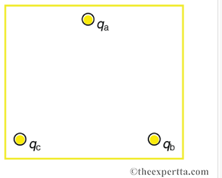# Problem: In the figure, the point charges are located at the corners of an equilateral triangle 26 cm on a side.Find the magnitude of the electric field, in newtons per coulomb, at the center of the triangular configuration of charges, given that qa = 1.9 nC, qb = -8.7 nC, and qc = 1.5 nC.

###### FREE Expert Solution

Electric field:

$\overline{){\mathbf{E}}{\mathbf{=}}\frac{\mathbf{k}\mathbf{q}}{{\mathbf{r}}^{\mathbf{2}}}}$

a = 26cm

r, the distance between the point at the center to one of the charges is given by, r = a/sqrt(3) = 15.0 cm

$\begin{array}{rcl}{\mathbf{E}}_{\mathbf{a}}& \mathbf{=}& \frac{\mathbf{\left(}\mathbf{9}\mathbf{×}{\mathbf{10}}^{\mathbf{9}}\mathbf{\right)}\mathbf{\left(}\mathbf{1}\mathbf{.}\mathbf{9}\mathbf{×}{\mathbf{10}}^{\mathbf{-}\mathbf{9}}\mathbf{\right)}}{{\mathbf{\left(}\mathbf{15}\mathbf{×}\mathbf{10}\mathbf{-}\mathbf{2}\mathbf{\right)}}^{\mathbf{2}}}\mathbf{=}\mathbf{760}\mathbf{N}\mathbf{/}\mathbf{C}\\ {\mathbf{E}}_{\mathbf{b}}& \mathbf{=}& \frac{\mathbf{\left(}\mathbf{9}\mathbf{×}{\mathbf{10}}^{\mathbf{9}}\mathbf{\right)}\mathbf{\left(}\mathbf{-}\mathbf{8}\mathbf{.}\mathbf{7}\mathbf{×}{\mathbf{10}}^{\mathbf{-}\mathbf{9}}\mathbf{\right)}}{{\mathbf{\left(}\mathbf{15}\mathbf{×}{\mathbf{10}}^{\mathbf{-}\mathbf{2}}\mathbf{\right)}}^{\mathbf{2}}}\mathbf{=}\mathbf{-}\mathbf{3480}\mathbf{N}\mathbf{/}\mathbf{C}\\ {\mathbf{E}}_{\mathbf{c}}& \mathbf{=}& \frac{\mathbf{\left(}\mathbf{9}\mathbf{×}{\mathbf{10}}^{\mathbf{9}}\mathbf{\right)}\mathbf{\left(}\mathbf{1}\mathbf{.}\mathbf{5}\mathbf{×}{\mathbf{10}}^{\mathbf{-}\mathbf{9}}\mathbf{\right)}}{{\mathbf{\left(}\mathbf{15}\mathbf{×}{\mathbf{10}}^{\mathbf{-}\mathbf{2}}\mathbf{\right)}}^{\mathbf{2}}}\mathbf{=}\mathbf{600}\mathbf{N}\mathbf{/}\mathbf{C}\end{array}$

92% (269 ratings)###### Problem Details

In the figure, the point charges are located at the corners of an equilateral triangle 26 cm on a side.
Find the magnitude of the electric field, in newtons per coulomb, at the center of the triangular configuration of charges, given that qa = 1.9 nC, qb = -8.7 nC, and qc = 1.5 nC.